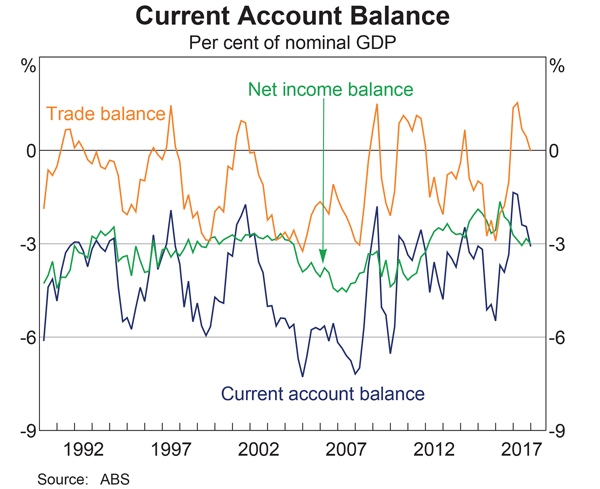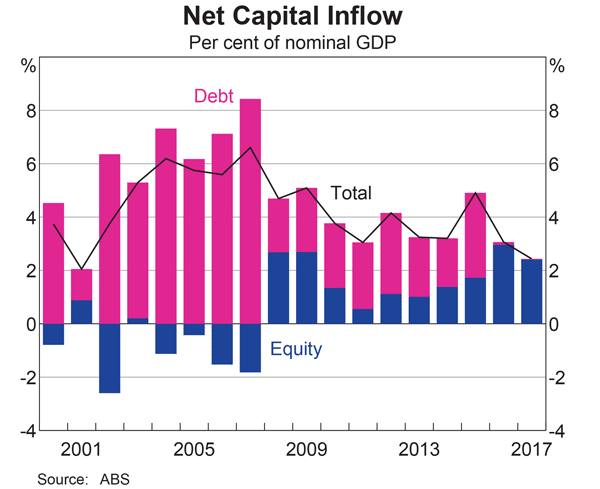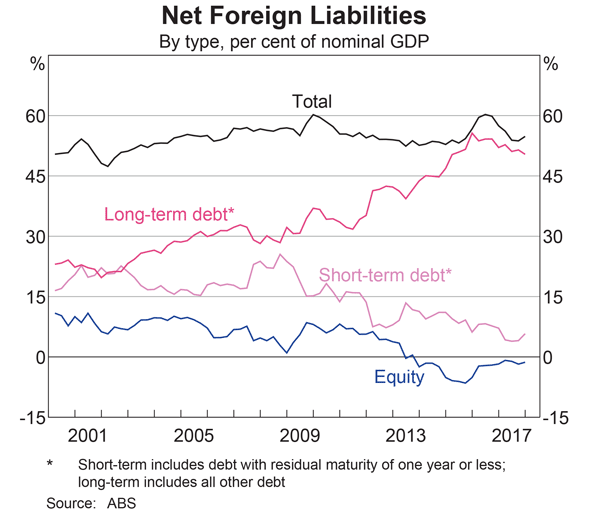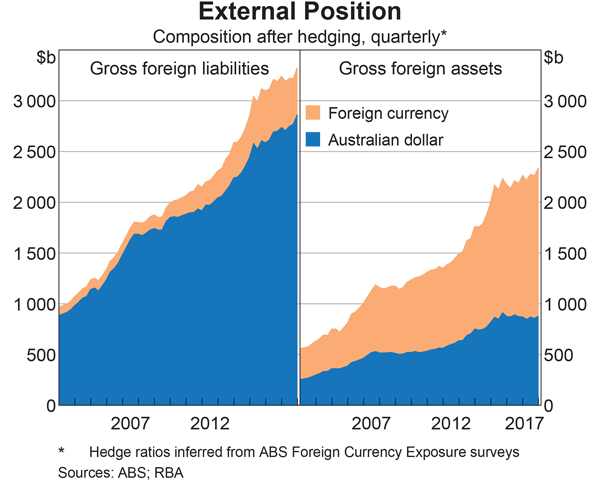Fight Finance

CoursesTagsRandomAllRecentScores

A firm can issue 3 year annual coupon bonds at a yield of 10% pa and a coupon rate of 8% pa.

The beta of its levered equity is 2. The market's expected return is 10% pa and 3 year government bonds yield 6% pa with a coupon rate of 4% pa.

The market value of equity is $1 million and the market value of debt is$1 million. The corporate tax rate is 30%.

What is the firm's after-tax WACC? Assume a classical tax system.

You operate a cattle farm that supplies hamburger meat to the big fast food chains. You buy a lot of grain to feed your cattle, and you sell the fully grown cattle on the livestock market.

You're afraid of adverse movements in grain and livestock prices. What options should you buy to hedge your exposures in the grain and cattle livestock markets?

Select the most correct response:

A zero coupon bond that matures in 6 months has a face value of $1,000. The firm that issued this bond is trying to forecast its income statement for the year. It needs to calculate the interest expense of the bond this year. The bond is highly illiquid and hasn't traded on the market. But the finance department have assessed the bond's fair value to be$950 and this is its book value right now at the start of the year.

Assume that:

• the firm uses the 'effective interest method' to calculate interest expense.
• the market value of the bond is the same as the book value.
• the firm is only interested in this bond's interest expense. Do not include the interest expense for a new bond issued to refinance the current one, as would normally happen.

What will be the interest expense of the bond this year for the purpose of forecasting the income statement?

A firm wishes to raise $8 million now. They will issue 7% pa semi-annual coupon bonds that will mature in 10 years and have a face value of$100 each. Bond yields are 10% pa, given as an APR compounding every 6 months, and the yield curve is flat.

How many bonds should the firm issue?

A stock's standard deviation of returns is expected to be:

• 0.09 per month for the first 5 months;
• 0.14 per month for the next 7 months.

What is the expected standard deviation of the stock per year $(\sigma_\text{annual})$?

Assume that returns are independently and identically distributed (iid) and therefore have zero auto-correlation.

Which one of the following will have no effect on net income (NI) but decrease cash flow from assets (CFFA or FFCF) in this year for a tax-paying firm, all else remaining constant?

Remember:

$$NI=(Rev-COGS-FC-Depr-IntExp).(1-t_c )$$ $$CFFA=NI+Depr-CapEx - ΔNWC+IntExp$$

Where can a publicly listed firm's book value of equity be found? It can be sourced from the company's:

Where can a private firm's market value of equity be found? It can be sourced from the company's:

The below table summarises the borrowing costs confronting two companies A and B.

 Bond Market Yields Fixed Yield to Maturity (%pa) Floating Yield (%pa) Firm A 3 L - 0.4 Firm B 5 L + 1

Firm A wishes to borrow at a floating rate and Firm B wishes to borrow at a fixed rate. Design an intermediated swap (which means there will actually be two swaps) that nets a bank 0.1% and shares the remaining swap benefits between Firms A and B equally. Which of the following statements about the swap is NOT correct?

Below are four graphs of Australia's external position, published in the RBA's chart pack.Assume that all assets and liabilities are debt which makes interest income and equity which makes dividend income.

Which of the following statements is NOT correct? As at the end of 2017, Australia’s: